Latest Banking jobs   »

# Quantitative Aptitude Quiz For Bank Foundation 2023 – 22nd March

Q1. Difference between 40% of y and 20% of x is 270 whereas difference between 40% of x and 20% of y is zero. Find the sum of ‘x’ and ‘y’ ?
(a) 1250
(b)1400
(c) 1200
(d) 1350
(e)1500
Q2. In an election between P and Q , if 2/5 th of the total voters promised to vote for P and rest promised to vote for Q. On the voting day 25% of the voters went back on their promise to vote for P & 30% of the voters went back on their promise to vote for Q. Find the total no. of voters, if Q wins by 400 votes.
(a) 8000
(b) 10000
(c) 15000
(d) 5000
(e) 12000
Q3. Present age of two friends, Veer and Rohit is in the ratio of 7 : 5. Arun is 10 years elder than Rohit. 10 years ago ratio of age of Veer and Arun is 16 : 15. Find the present age of Rohit.
(a) 42 years
(b) 40 years
(c) 30 years
(d) 20 years
(e) 28 years

Q4. Seven years ago ratio of age of Abhi and Rahul is 5 : 3 and 8 years hence their ratio of ages are 3 : 2. Find the age of Sati whose present age is equal to average of present age of Abhi and Rahul ?
(a) 53 years
(b) None of these
(c) 58 years
(d) 63 years
(e) 67 years

Q5. Mohan was married 5 years ago. At present his age is 6/5 times of his age at the time of marriage. His wife is 3 years younger to him. Find his wife’s present age. (in years)
(a) 22
(b) 27
(c) 21
(d) 24
(e) None of these

Q6. Aman’s age is twice the Rahul’s age who is 5 years older than Arun. If the total age of Rahul, Aman and Arun is 79. Then find the difference between Aman’s age and Arun’s age.
(a) 30 years
(b) 19 years
(c) 26 years
(d) 16 years
(e) 35 years
Q7. a, b, c and d are four consecutives even numbers, if the sum of ‘a’ and ‘c’ is 120, what is the product of ‘b’ and ‘d’?
(a) 4030
(b) 3780
(c) 3900
(d) 3750
(e) 3840

Q8. Three numbers are given. The average of first and third numbers is 24 more than that of average of second and third numbers. Find out the difference between the first and second numbers.
(a) 36
(b) 40
(c) 42
(d) 48
(e) 46
Q9. The sum of digits of a two-digit number is 12 and the difference between the two-digits of the two-digit number is 6. What is the two-digit number?
(a) 39
(b) 28
(c) 93
(d) 75
(e) Either (a) or (c)

Q10. Present average age of a couple is 29 years after 2 year and 4 year respectively a boy child and a girl child is born. Find the average age of family after 8 year of present time? (in years)
(a) 18
(b) 19
(c) 20
(d) 21
(e) 22

Q11. 2/3rd of first number is equal to the cube of the second number. If the second number is equal to 12% of 100, what is sum of the first & second number?
(a) 2408
(b) 2640
(c) 2426
(d) 2604
(e) 2804

Q12. The present age of Bhagat and Abhi are in ratio of 9 : 8 respectively. After 10 years the respective ratio of their ages will be 10 : 9. What is the difference in their present age ?
(a) 8 years
(b) 6 years
(c) 12 years
(d) 4 years
(e) 10 years

Q13. If income & saving ratio of a person is 25 : 9. If saving increases 1/3rd & expenditure increases by 25% & income increases by Rs. 2100. Then find the initial saving?
(a) Rs. 3200
(b) Rs. 3500
(c) Rs. 5600
(d) Rs. 2700
(e) Rs. 4200

Q14. Sum of four consecutive even numbers is 49 more than sum of three consecutive odd numbers and the sum of lowest odd and lowest even number is 23. Find the largest even number.
(a) 28
(b) 36
(c) 18
(d) 32
(e) 22
Q15. 3/25 students who registered in an exam did not appeared in the exam while 5/9 student who appeared were failed in the exam. If 1800 is total number of students who registered then, find the number of students who passed the exam?
(a) 120
(b) 96
(c) 704
(d) 880
(e) 780

Solutions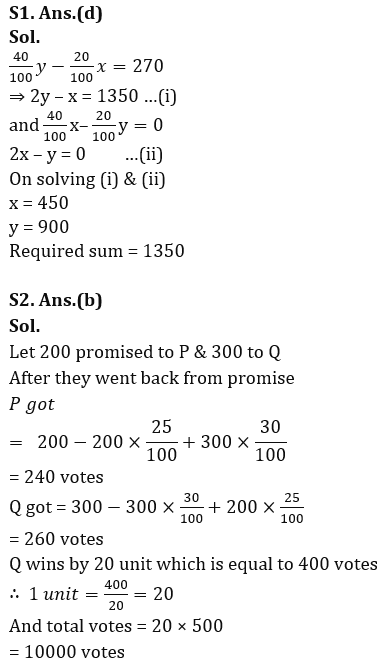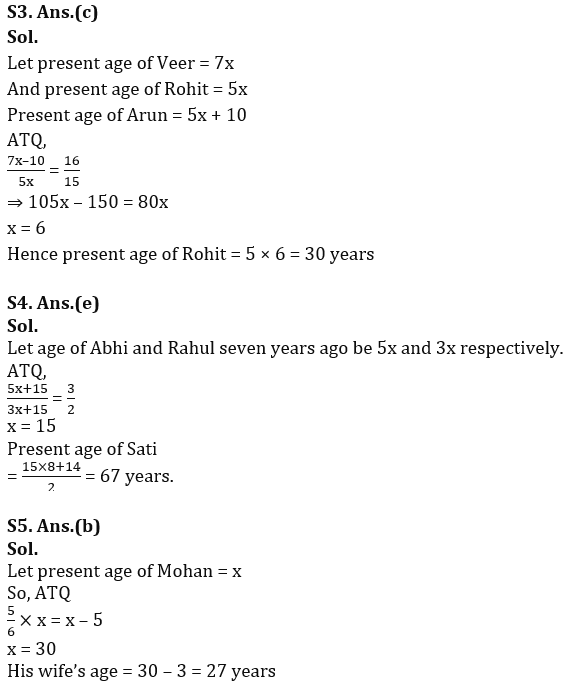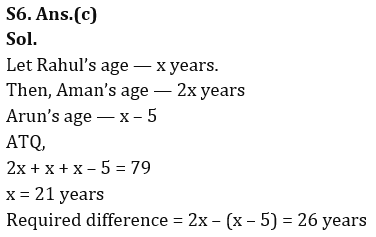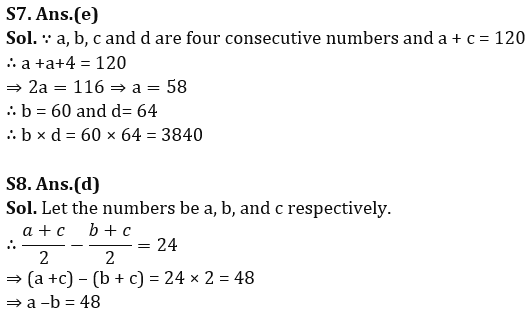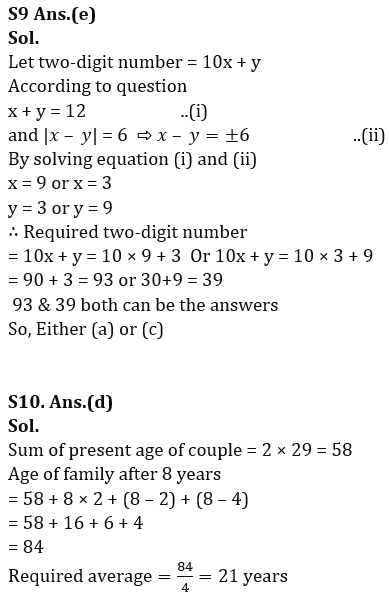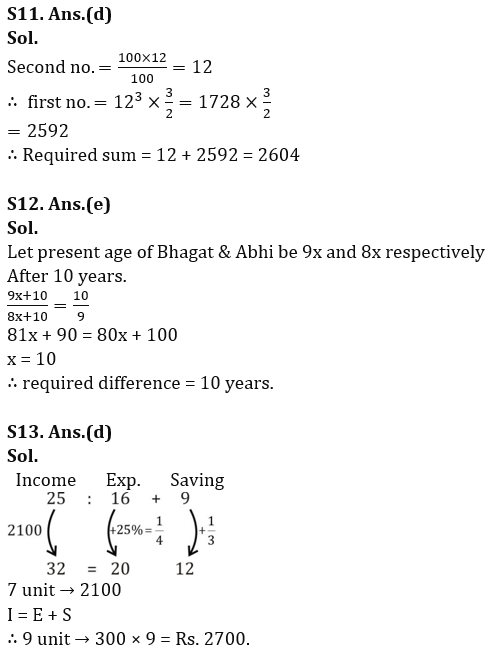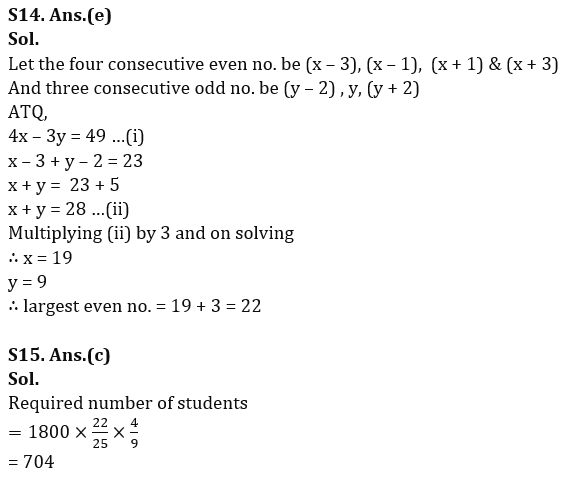## FAQs

### What is the selection process of the Bank Clerk?

The selection process of the Bank Clerk is Prelims & Mains.

#### Congratulations!Union Budget 2023-24: Free PDF